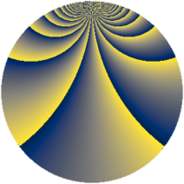Properties

 Label 432.2.beLevel $432$ Weight $2$ Character orbit 432.be Rep. character $\chi_{432}(47,\cdot)$ Character field $\Q(\zeta_{18})$ Dimension $108$ Newform subspaces $3$ Sturm bound $144$ Trace bound $11$

Related objects

Defining parameters

 Level: $$N$$ $$=$$ $$432 = 2^{4} \cdot 3^{3}$$ Weight: $$k$$ $$=$$ $$2$$ Character orbit: $$[\chi]$$ $$=$$ 432.be (of order $$18$$ and degree $$6$$) Character conductor: $$\operatorname{cond}(\chi)$$ $$=$$ $$108$$ Character field: $$\Q(\zeta_{18})$$ Newform subspaces: $$3$$ Sturm bound: $$144$$ Trace bound: $$11$$ Distinguishing $$T_p$$: $$5$$, $$7$$

Dimensions

The following table gives the dimensions of various subspaces of $$M_{2}(432, [\chi])$$.

Total New Old
Modular forms 468 108 360
Cusp forms 396 108 288
Eisenstein series 72 0 72

Trace form

 $$108 q + O(q^{10})$$ $$108 q + 36 q^{29} + 18 q^{33} + 18 q^{41} + 36 q^{45} - 18 q^{57} - 72 q^{65} - 72 q^{69} - 72 q^{77} - 72 q^{81} - 54 q^{89} - 72 q^{93} + 54 q^{97} + O(q^{100})$$

Decomposition of $$S_{2}^{\mathrm{new}}(432, [\chi])$$ into newform subspaces

Label Dim. $$A$$ Field CM Traces $q$-expansion
$a_{2}$ $a_{3}$ $a_{5}$ $a_{7}$
432.2.be.a $36$ $3.450$ None $$0$$ $$0$$ $$-6$$ $$0$$
432.2.be.b $36$ $3.450$ None $$0$$ $$0$$ $$3$$ $$0$$
432.2.be.c $36$ $3.450$ None $$0$$ $$0$$ $$3$$ $$0$$

Decomposition of $$S_{2}^{\mathrm{old}}(432, [\chi])$$ into lower level spaces

$$S_{2}^{\mathrm{old}}(432, [\chi]) \cong$$ $$S_{2}^{\mathrm{new}}(108, [\chi])$$$$^{\oplus 3}$$